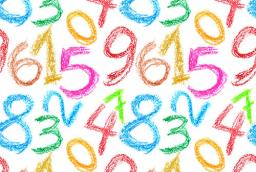# Multiplied 4545

Philip multiplied the number 4 twice in a row by his lucky number. He added 4 to the result and got a result of 200. What is Filip's lucky number?

x1 =  7
x2 =  -7

### Step-by-step explanation:Did you find an error or inaccuracy? Feel free to write us. Thank you!

Tips for related online calculators
Are you looking for help with calculating roots of a quadratic equation?
Do you have a linear equation or system of equations and looking for its solution? Or do you have a quadratic equation?